# 叶片粗糙度对压气机性能影响研究 Study on the Influence of Blade Roughness on Compressor Performance

• 全文下载: PDF(1848KB)    PP.365-372   DOI: 10.12677/APP.2019.98043
• 下载量: 141  浏览量: 231

There are many factors that affect the performance of aero-engine, among which the change of compressor blade surface roughness caused by scaling is the representative one. The change of blade surface roughness directly affects the change of effective flow area of air passage, and then causes the change of supercharging capacity, efficiency and flow capacity. In this paper, the flow field simulation technique is used to study the performance variation of compressor under different blade roughness. The results show that when the blade roughness decreases, the compressor boost ratio and efficiency increase significantly, the shock wave intensity on the blade surface decreases, the total temperature decreases, and the overall performance of the compressor improves significantly.

1. 引言

2. 数值办法

$\left\{\begin{array}{l}\frac{\partial \rho }{\partial t}+\frac{\partial }{\partial {x}_{i}}\left(\rho {u}_{i}\right)=0\\ \frac{\partial }{\partial t}\left(\rho {u}_{i}\right)+\frac{\partial }{\partial {x}_{j}}\left(\rho {u}_{i}{u}_{j}\right)+\frac{\partial p}{\partial {x}_{i}}=\frac{\partial }{\partial {x}_{j}}\left[{\mu }_{eff}\left(\frac{\partial {u}_{j}}{\partial {x}_{i}}+\frac{\partial {u}_{i}}{\partial {x}_{j}}\right)\right]-\frac{2}{3}\frac{\partial }{\partial {x}_{j}}\left[{\mu }_{eff}\left(\frac{\partial {u}_{i}}{\partial {x}_{i}}\right)\right]\\ \frac{\partial }{\partial t}\left(\rho E\right)+\frac{\partial }{\partial {x}_{i}}\left(\rho {u}_{i}E\right)+\frac{\partial p{u}_{i}}{\partial {x}_{i}}=\frac{\partial }{\partial {x}_{j}}\left[{\mu }_{eff}{u}_{i}\left(\frac{\partial {u}_{j}}{\partial {x}_{i}}+\frac{\partial {u}_{i}}{\partial {x}_{j}}\right)\right]-\frac{2}{3}\frac{\partial }{\partial {x}_{j}}\left[{\mu }_{eff}{u}_{i}\left(\frac{\partial {u}_{i}}{\partial {x}_{i}}\right)\right]+\frac{\partial }{\partial {x}_{j}}\left[{k}_{eff}\left(\frac{\partial T}{\partial {x}_{j}}\right)\right]\\ p=\rho RT\end{array}$ (1)

$\frac{\partial \left(\mathcal{l}k\right)}{\partial t}+\frac{\partial \left(\mathcal{l}k{u}_{i}\right)}{\partial {x}_{i}}=\frac{\partial }{\partial {x}_{j}}\left[\left(\mu +\frac{{\mu }_{t}}{{\sigma }_{t}}\right)\frac{\partial k}{\partial {x}_{j}}\right]+{G}_{k}+{G}_{b}-\mathcal{l}e-{Y}_{M}+{S}_{k}$ (2)

e方程是个由经历公式导出的方程：

$\frac{\partial \left(\mathcal{l}e\right)}{\partial t}+\frac{\partial \left(\mathcal{l}e{u}_{i}\right)}{\partial {x}_{i}}=\frac{\partial }{\partial {x}_{j}}\left[\left(\mu +\frac{{\mu }_{t}}{{\sigma }_{e}}\right)\frac{\partial e}{\partial {x}_{j}}\right]+{C}_{1}e\frac{e}{k}\left({G}_{k}+{C}_{3}e{G}_{b}\right)-{C}_{2}\mathcal{l}\frac{{e}^{2}}{k}+{S}_{e}$ (3)

3. 粗糙度的描述

1) 近于滑腻壁面： $0\text{μm}\le {h}_{s}\le 5\text{μm}$

2) 过渡粗糙壁面： $5\text{μm}\le {h}_{s}\le 70\text{μm}$

3) 完全粗糙壁面： ${h}_{s}>70\text{μm}$Figure 1. Sand roughness model

${u}^{+}=1×\mathrm{ln}\left[{y}^{+}/\left(1+0.3×{k}^{+}\right)\right]/k+C$ (4)

${k}^{+}={y}_{R}×\rho ×{u}^{+}/\mu$ (5)

4. 模型的建立与求解

4.1. 模型建立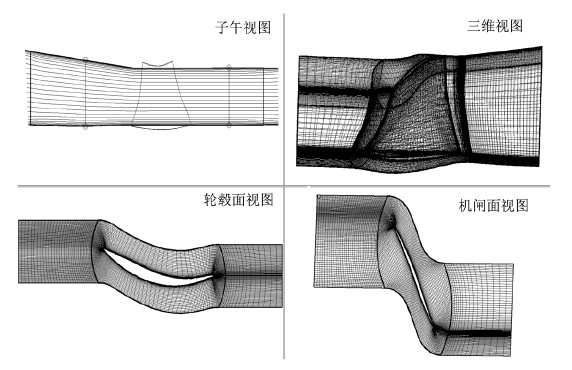Figure 2. View of blade profile

4.2. 仿真计算

5. 仿真成果与分析Figure 3. The effect of different roughness on compressor characteristics at 100% rotating speedFigure 4. The effect of different roughness on compressor characteristics at 90% rotating speed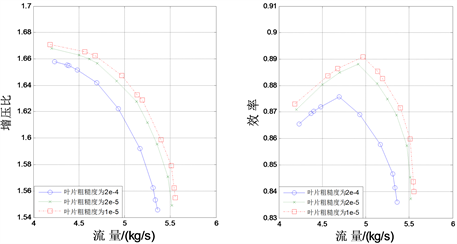Figure 5. The effect of different roughness on compressor characteristics at 80% rotating speed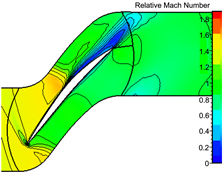(a) ks = 2e−4(b) ks = 2e−5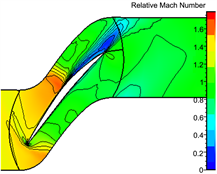(c) ks = 1e−5

Figure 6. The influence of different roughness on shock wave on blade surface when rotating speed is 100%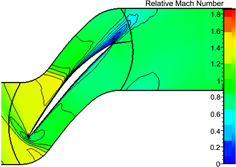(a) ks = 2e−4(b) ks = 2e−5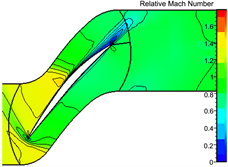(c) ks = 1e−5

Figure 7. The influence of different roughness on the shock wave on blade surface when rotating speed is 90%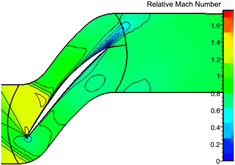(a) ks = 2e−4(b) ks = 2e−5(c) ks = 1e−5

Figure 8. The influence of different roughness on the shock wave on blade surface when rotating speed is 80%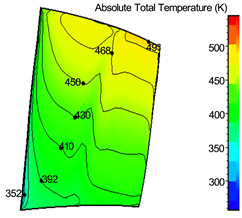(a) ks = 2e−4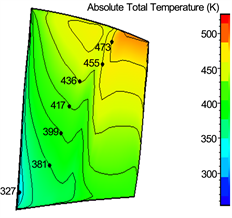(b) ks = 2e−5(c) ks = 1e−5

Figure 9. The influence of different roughness on the total temperature on blade surface when rotating speed is 100%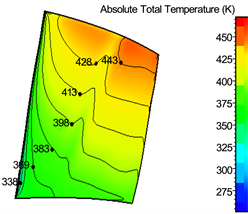(a) ks = 2e−4(b) ks = 2e−5(c) ks = 1e−5

Figure 10. The influence of different roughness on the total temperature on blade surface when rotating speed is 90%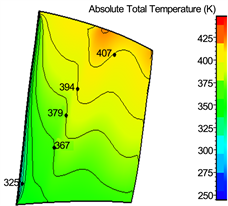(a) ks = 2e−4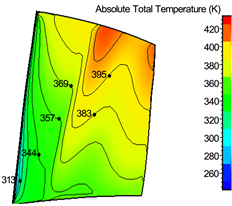(b) ks = 2e−5(c) ks = 1e−5

Figure 11. The influence of different roughness on the total temperature on blade surface when rotating speed is 80%

6. 结论

1) 叶片外面粗糙度的降低，这将招致压气机增压比和效力明显进步，扩大年夜了压气机稳定任务范围，改良压气机整体任务特点，进步了压气机本身做功的才能，增添了活动损掉。

2) 随着叶片外面粗糙度的降低，吸力面侧激波的地位向叶片出口偏向逐步移动，且强度减小明显，进一步使得叶片的尾迹区范围减小。

3) 随着叶片外面粗糙度的降低，叶片外面总温有不合程度的降低。当叶片粗糙度降低后，叶片外面总温的降低幅度从叶片底部到叶片顶部顺次减小，随着转速降低顺次减小。

  刘大年夜响. 航空发动机[M]. 北京: 航空工业出版社, 2008.  孙护国. 涡轴发动机清洗技巧及其生长[C]//中国航空学会. 第十六届全国直升机年会论文集. 北京: 北京航空航天大年夜学出版社, 2008.  Back, S.C., Sohn, J.H. and Song, S.J. (2010) Impact of Surface Roughness on Compressor Cascade Performance. Journal of Fluids Engi-neering, 132, Article ID: 064502.  Millsaps, K.T. (2012) Effects of Reynolds Number and Surface Roughness Magnitude and Location on Compressor Cascade Performance. Journal of Turbomachinery, 134, Article ID: 051013. https://doi.org/10.1115/1.4003821  Suder, K.L., Chima, R.V., Strazisar, A.J. and Roberts, W.B. (1995) The Effect of Adding Roughness and Thickness to a Transonic Axial Compressor Rotor. ASME Journal of Turbomachinery, 117, 491-505. https://doi.org/10.1115/1.2836561  李本威, 李冬, 李姜华, 等. 单级压气机性能阑珊定量研究[J]. 航空动力学报, 2010, 25(7): 1588-1594.  李钊, 李本威, 王东艺, 等. 压气机性能参数对积垢敏理性分析[J]. 航空计算技巧, 2011, 41(6): 41-44+48.  郑贇, 田晓, 杨慧. 跨声速电扇叶片变形对气动性能的影响[J]. 航空动力学报, 2011, 26(7): 1621-1627.  陈绍文, 张辰, 石慧, 等. 轴流压气机污垢堆积影响的数值研究[J]. 推动技巧, 2012, 33(3): 377-383.  尹松, 金东海, 朱芳, 等. 湍流模型对压气机数值模仿精度的影响[J]. 航空动力学报, 2010, 25(12): 2683-2689.  颜青钦. 涡扇发动机电扇性能阑珊机理及其影响规律研究[D]: [硕士学位论文]. 烟台: 海军航空工程学院, 2012.  李绍斌, 苏杰先, 冯国泰, 等. 动态叶片搅扰下跨声压气机级性能与叶片型面静压研究[J]. 航空动力学报, 2007, 22(7): 1153-1160.  李本威, 李冬, 沈伟, 等. 涡轮叶片粗糙度对其性能阑珊的影响研究[J]. 航空计算技巧, 2009, 39(5): 26-29+34.